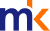Most CommentedFaster to learn maths and science in Chinese

Does anyone knows that why Chinese school students are good in mathematics and science? One major reason is because of the language. Mandarin is a more efficient language than other language. Here are the explanations.

To count 1 to 10 in mandarin, it takes 10 vocals. To count 1 to 10 in English, it takes 11 vocals because ‘seven’ has two vocals. To count 1 to 10 in Bahasa Malaysia, it takes 22 vocals. e.g. 1: sa-tu (two vocals), 9: sem-bi-lan (three vocals).

For the same person to solve one mathematical problem, he spends less time to calculate when using Mandarin compared to Bahasa Malaysia.

Example 1:

2 - 1 = 1

In Mandarin, 'er jian yi tang yu yi' (six vocals)

In English, 'two mi-nus one e-qu-als to one' (nine vocals)

In Bahasa, 'Du-a to-lak sa-tu sa-ma de-ngan sa-tu' (12 vocals)

Example 2 :

2 x 2 = 4

In Mandarin, ‘er cheng er tang yu si’ (six vocals)

In English, ‘two times two e-qu-als to four’ (eight vocals)

In Bahasa, ‘Du-a ka-li du-a sa-ma de-ngan em-pat’ (12 vocals)

To learn mathematics in Mandarin, it would take half the time compared to Bahasa Malaysia. Mathematics is a subject where 'practice makes perfect'. Within the same period of time, solving mathematical problems in Mandarin becomes more faster when compared to using Bahasa.

For the one hour of a mathematics examination, a Chinese-educated student may use 40 minutes of the time to answer all the questions and spends another 20 minutes checking his answers. While a Bahasa-educated student may not able to answer all the questions within the one hour allotted.

Science, meanwhile, requires a good memory and imagination. ‘Han Ji’ are symbolic and pictorial characters. There are more than thousand of these Chinese characters.

To learn Chinese, a student is indirectly trained to memorise and this thus stimulates the brain cells for memory and imagination. Hence, from the learning of Chinese, indirectly, students are trained up in the field of mathematics and science.